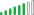cancel
Showing results for
Did you mean:Helper III

## DAX Calculate Percentage from Column Total

I am trying to build a tabular cube measure that will always calculate the percentage from the column total even when using a matrix.In the example attached i would like to get X % of Total and Y % of Toal columns.
The measure should be dynamic and work with regardless of the dimension the user will place in the columns so the option of using Calculate(SUM(Salse_Quantity),ALL(Products[Product Name])) or                    Calculate(SUM(Salse_Quantity),ALLSELECTED(Products)) is not good enough.

Is there any way to achieve this?

Thanks!Solution Supplier

You cannot detect what is on rows or what is on columns in DAX, so you cannot do a true "Column Total". However, something like this, may work for you:

SUM(Salse_Quantity) /

Calculate(SUM(Salse_Quantity)
,Product
,ALLSELECTED()
)